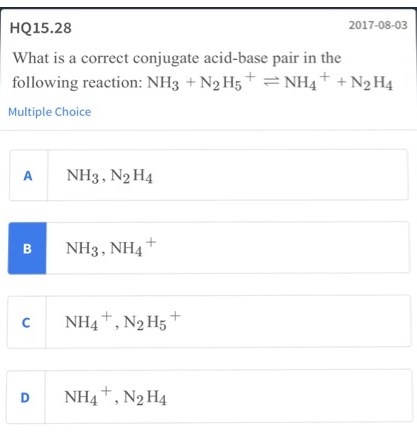Chemistry Practice Problems Conjugate Acids and Bases Practice Problems Solution: What is a correct conjugate acid-base pair in the ...

🤓 Based on our data, we think this question is relevant for Professor Mollica's class at BROOKLYN CUNY.

# Solution: What is a correct conjugate acid-base pair in the following reaction: NH3 + N2H5+ ⇌ NH4+ + N2H4a. NH3, N2H4b. NH3, NH4+c. NH4+, N2H5+d. NH4+, N2H4

###### Problem

What is a correct conjugate acid-base pair in the following reaction: NH3 + N2H5+ ⇌ NH4+ + N2H4

a. NH3, N2H4

b. NH3, NH4+

c. NH4+, N2H5+

d. NH4+, N2HView Complete Written Solution

Conjugate Acids and Bases

Conjugate Acids and Bases

#### Q. What is the conjugate acid of HN2-?(a) N3-(b) H2N-(c) H3N(d) HN2-(e) H4N+(f) None of these choices is correct.

Solved • Mon Oct 01 2018 15:52:08 GMT-0400 (EDT)

Conjugate Acids and Bases

#### Q. What is the correct conjugate acid-base pair in the following reaction: HNO3 + N2H4 ⇌ NO3- + N2H5+a. HNO3, N2H4b. N2H4, NO3-c. N2H4, N2H5+d. HNO3, N2H...

Solved • Mon Jul 30 2018 17:15:09 GMT-0400 (EDT)

Conjugate Acids and Bases

#### Q. In the following reaction, HCO3- (aq) + H2O (l) ⇌ CO32- (aq) + H3O+ (aq) a. H3O+ is an acid and HCO3- is its conjugate base. b. HCO3- is an acid and C...

Solved • Mon Jul 30 2018 11:38:33 GMT-0400 (EDT)

Conjugate Acids and Bases

#### Q. Write the formula of the conjugate acid to the following species regarded as a base: HASO42-.

Solved • Mon Jul 02 2018 15:50:49 GMT-0400 (EDT)